# Force

Physics is the science that studies motion and matter and how both work with forces and energy.

It is a really big subject that has a lot of different “branches” of scientific study that include motion, sound, waves, electricity, light, sound, and even astronomy. This science covers the tiniest particles and atoms all the way to the largest objects in the universe.

When we talk about “force” in physics, we are speaking of something that is a pull or push on an object to cause that object to accelerate, slow down, change shape, or remain in place.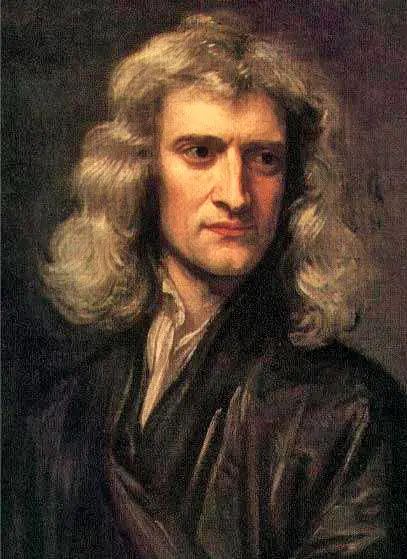Sir Isaac Newton assigned the abbreviation of “N” for “newton” as the unit of measurement for force in the mathematical formulas. One “newton” is the amount of force required to accelerate one gram of mass by one centimeter per second, squared. There are other units of force that include the “dyne” and the “pound-force.”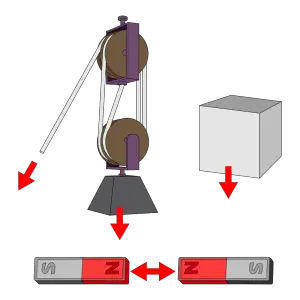### Types of Forces

Contact force is the type of force when two objects interact or have physical contact with each other. This can include frictional, tension, normal, air resistance, applied and spring forces.

• Friction is a type of force that is caused when one object is rubbing against another object. It works in the opposing direction of the main force.
• Normal force is a kind of force that is exerted on an object that is in contact with a difference object that is stable. An example might be a person leaning against a wall.
• Air resistance force is a kind of frictional force for objects in the air. They air resistance force opposes the movement of the object itself. An example would be a parachute or airplane.
• Applied force is when an object has been pulled or pushed. An example might be pushing a table to another side of the room.
• Gravity is the force caused by a large body, such as our Earth, that pulls other objects towards it.
• Electromagnetic force is part of the effects from electric and magnetic fields.
• Nuclear forces are those that are involved in holding atoms and their particles together.
• Tension is a kind of force involved in pulling that is exerted on another object such as a cable or string.
• Elastic force is the kind of force exerted by an object as it tries to return to its natural length after having been pushed or pulled by an external force.

### Force is a vector

We call force a “vector” because it has a direction. The “magnitude” of vectors is the measurement from the start or head to the stop or tail.

### Equilibrium Forces

Sometimes there can be multiple forces that are acting on an object that ends up making it stay still. An example of this might be two teams playing tug of war, each team pulling the same so that the knot in the center never ends up on one side or the other. The sum of the forces in this case, or “net force” is zero. The forces are in “equilibrium.”

### Combined Forces

This is a situation where the combined forces that are acting on an object results in the sum of the vectors of each of the forces. Let’s use the same tug of war example: 1 team is pulling their left side at 3N and the other team is pulling their right side at 4N. The resulting force ends up with 1N for the right side (and they would win.)

### Effecting Force:

There are different actions and other forces that can influence the velocity of an object. Thrust is a force that increases an object’s velocity and drag is the force that decreases its velocity.

When an object is increasing acceleration in a circular motion it experiences what is called “centripetal” force.

### Q&A:

What does the “N” stand for in the unit of measurement for force?
newton

What type of force creates a “net zero force”?
equilibrium

What type of force does an airplane flying in the air exhibit?
air resistance force

What is the definition of “force” in physics?
something that is a pull or push on an object to cause that object to accelerate, slow down, change shape, or remain in place

When one team wins in a tug of war, what kind of force are they participating in?
combined force

•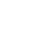History
•Science
•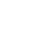Geography
•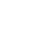Biography
•US History